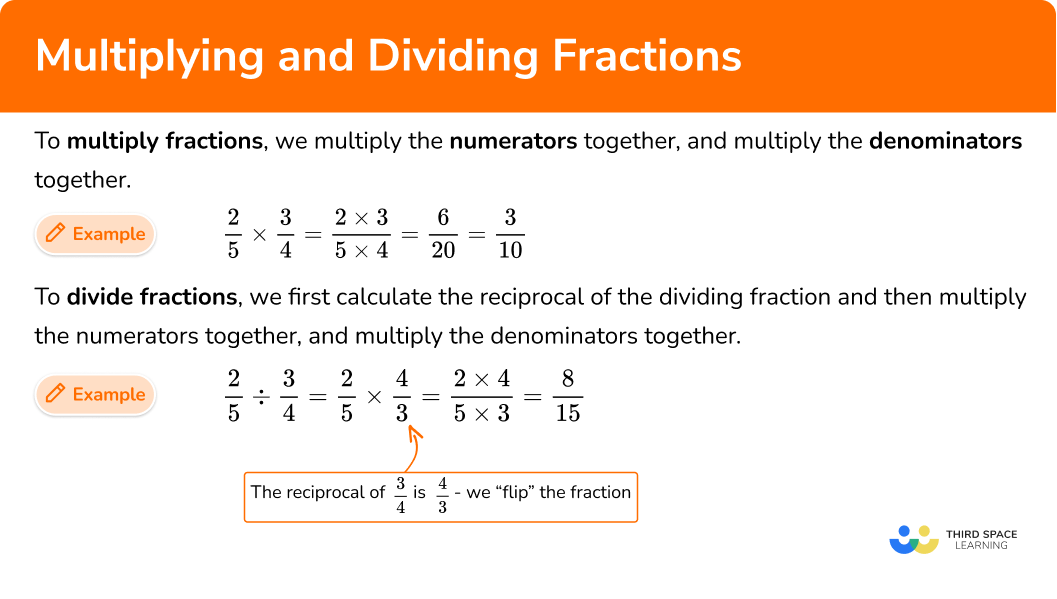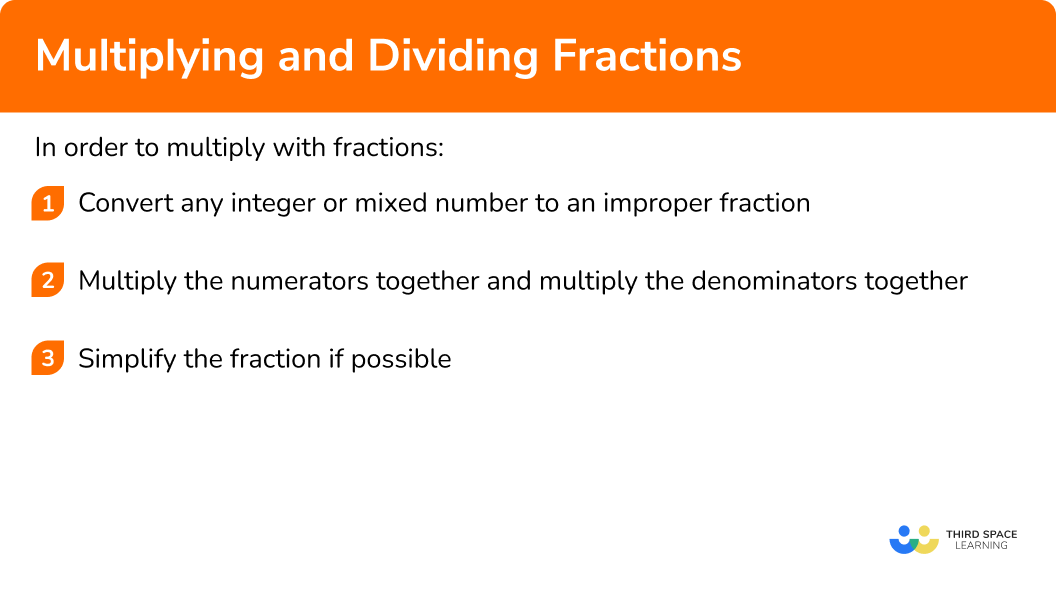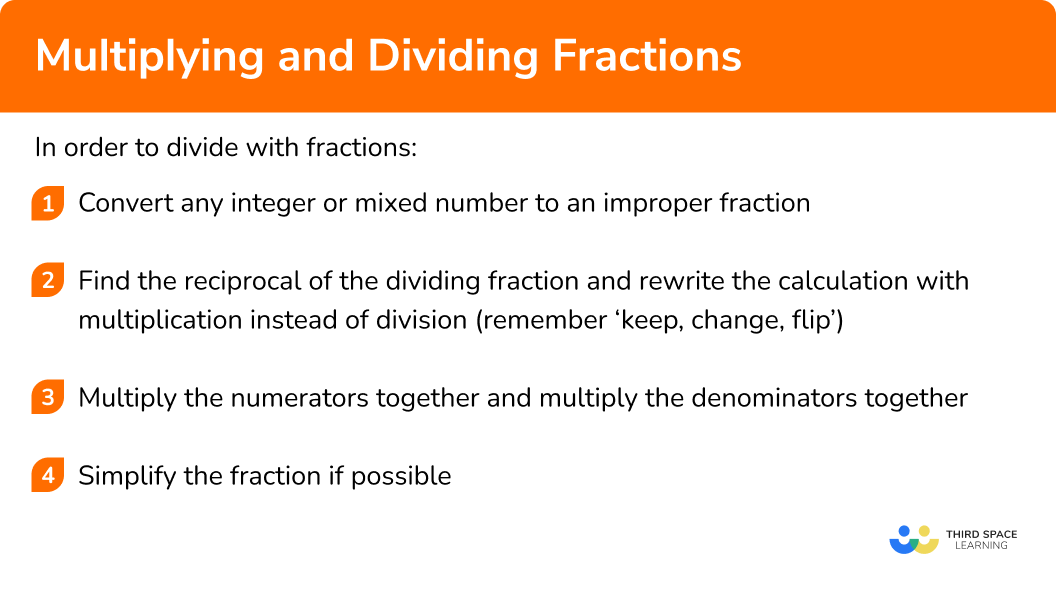GCSE Maths Number FDP Fractions

Multiplying And Dividing Fractions

# Multiplying And Dividing Fractions

Here we will learn about multiplying and dividing fractions, including calculations involving whole numbers, proper and improper fractions, and mixed numbers.

There are also multiplying and dividing fractions worksheets based on Edexcel, AQA and OCR exam questions, along with further guidance on where to go next if you’re still stuck.

## What is multiplying and dividing fractions?

Multiplying and dividing fractions is the skill of carrying out a calculation involving multiplication and division where one or more of the values is written as a fraction.

To multiply fractions, we multiply the numerators together, and multiply the denominators together.

For example,

\frac{2}{5} \times \frac{3}{4}=\frac{2 \times 3}{5 \times 4}=\frac{6}{20}=\frac{3}{10}

To divide fractions, we first calculate the reciprocal of the dividing fraction and then multiply the numerators together, and multiply the denominators together.

For example,

\frac{2}{5} \div \frac{3}{4}=\frac{2}{5} \times \frac{4}{3}=\frac{2 \times 4}{5 \times 3}=\frac{8}{15}

Note that, unlike when we add and subtract fractions, we do not need a common denominator to multiply and divide fractions.

### What is multiplying and dividing fractions?### Dividing by a fraction – in more detail

When we divide a quantity by a fraction, this is the same as multiplying by the reciprocal of this fraction. Let’s have a look at this diagrammatically.

For example, here is a circle, which has a value of 1.

Let’s divide this circle into halves (\div\frac{1}{2}).

Here we can see that the circle has been split into two halves.

This means that 1\div\frac{1}{2} is equal to 2.

Let’s look at another example, here are four squares.

If we wanted to divide these squares into two fifths (\div\frac{2}{5}), we would get,

If we total the number of two fifths in 4, we get 10.

This means that 4\div\frac{2}{5} is equal to 10.

How could we rearrange this division to become a multiplication instead?

Using 4\div\frac{2}{5}=10, as we are dividing 4 by two fifths, let’s multiply both sides by \frac{2}{5},

4=10\times\frac{2}{5}.

When multiplying a quantity by a fraction, we multiply the numerator and divide by the denominator and so if we split the fraction into these two operations, we have

4=10\times{2}\div{5}.

Rearranging this by using inverse operations, we need to multiply both sides by 5.

4\times{5}=10\times{2}

And then divide both sides by 2.

4\times{5}\div{2}=10

As 5\div{2} is the same as \frac{5}{2}, here we are now multiplying 4 by \frac{5}{2} or specifically,

4\times\frac{5}{2}=10.

Notice what has happened to the fraction. The fraction is now “upside down” meaning that we have found the reciprocal of the fraction.

The division has also changed now to be a multiplication.

This means that 4\div\frac{2}{5} gives us exactly the same result as 4\times\frac{5}{2}.

In general, any quantity divided by a fraction is identical to the same quantity being multiplied by the reciprocal of that fraction,

\frac{a}{b}\div\frac{c}{d}\equiv\frac{a}{b}\times\frac{d}{c}

## How to multiply with fractions

In order to multiply with fractions:

1. Convert any integer or mixed number to an improper fraction.
2. Multiply the numerators together and multiply the denominators together.
3. Simplify the fraction if possible.

### Explain how to multiply with fractions### Related lessons on fractions

Multiplying and dividing fractions is part of our series of lessons to support revision on fractions. You may find it helpful to start with the main fractions lesson for a summary of what to expect, or use the step by step guides below for further detail on individual topics. Other lessons in this series include:

## Multiplying and dividing fractions examples

### Example 1: multiplying an integer by a fraction

Determine the value of \ 3\times\frac{5}{7}.

1. Convert any integer or mixed number to an improper fraction.

The integer 3 can be written as the fraction \frac{3}{1}.

We now have the question \frac{3}{1}\times\frac{5}{7}.

2Multiply the numerators together and multiply the denominators together.

\frac{3}{1}\times\frac{5}{7}=\frac{3\times5}{1\times7}=\frac{15}{7}

3Simplify the fraction if possible.

\frac{15}{7}

This is an improper fraction which we should convert to a mixed number.

15\div 7 = 2 \ (remainder 1)

Therefore the mixed number has 2 as a whole number, and 1 remains to be divided by 7, giving 2\frac{1}{7}.

The solution is 2\frac{1}{7}.

### Example 2: multiplying two fractions

Determine the value of \ \frac{2}{5}\times\frac{3}{5}.

Convert any integer or mixed number to an improper fraction.

Multiply the numerators together and multiply the denominators together.

Simplify the fraction if possible.

### Example 3: multiplying three fractions

Determine the value of \ \frac{5}{8}\times\frac{2}{3}\times\frac{1}{6}.

Convert any integer or mixed number to an improper fraction.

Multiply the numerators together and multiply the denominators together.

Simplify the fraction if possible.

### Example 4: multiplying a fraction by a mixed number

Determine the value of \frac{1}{6}\times2\frac{1}{4}.

Convert any integer or mixed number to an improper fraction.

Multiply the numerators together and multiply the denominators together.

Simplify the fraction if possible.

## How to divide with fractions

In order to divide with fractions:

1. Convert any integer or mixed number to an improper fraction.
2. Find the reciprocal of the dividing fraction and rewrite the calculation with multiplication instead of division (remember ‘keep, change, flip’).
3. Multiply the numerators together and multiply the denominators together.
4. Simplify the fraction if possible.

### Explain how to divide with fractions### Example 5: dividing an integer by a fraction

Determine the value of \ 2\div\frac{3}{4}.

Convert any integer or mixed number to an improper fraction.

Find the reciprocal of the dividing fraction and rewrite the calculation with multiplication instead of division (remember ‘keep, change, flip’).

Multiply the numerators together and multiply the denominators together.

Simplify the fraction if possible.

### Example 6: dividing two fractions

Determine the value of \frac{1}{10}\div\frac{7}{10}.

Convert any integer or mixed number to an improper fraction.

Find the reciprocal of the dividing fraction and rewrite the calculation with multiplication instead of division (remember ‘keep, change, flip’).

Multiply the numerators together and multiply the denominators together.

Simplify the fraction if possible.

### Example 7: dividing a fraction by another fraction

Determine the value of \ \frac{3}{4}\div\frac{9}{10}.

Convert any integer or mixed number to an improper fraction.

Find the reciprocal of the dividing fraction and rewrite the calculation with multiplication instead of division (remember ‘keep, change, flip’).

Multiply the numerators together and multiply the denominators together.

Simplify the fraction if possible.

### Example 8: dividing a fraction by a mixed number

Determine the value of \ \frac{2}{3}\div{3}\frac{1}{5}.

Convert any integer or mixed number to an improper fraction.

Find the reciprocal of the dividing fraction and rewrite the calculation with multiplication instead of division (remember ‘keep, change, flip’).

Multiply the numerators together and multiply the denominators together.

Simplify the fraction if possible.

### Example 9: dividing a fraction by a whole number

Determine the value of \ \frac{3}{7}\div 5.

Convert any integer or mixed number to an improper fraction.

Find the reciprocal of the dividing fraction and rewrite the calculation with multiplication instead of division (remember ‘keep, change, flip’).

Multiply the numerators together and multiply the denominators together.

Simplify the fraction if possible.

### Common misconceptions

• Believing that there needs to be a common denominator in order to multiply or divide with fractions

When we add or subtract fractions there must be a common denominator. However, this is not a requirement when we multiply and divide with fractions.

When we multiply/divide with fractions they can have the same denominators or different denominators.

• Forgetting to write integers as fractions and then using a mathematically incorrect procedure

Sometime students make the following sort of mistake,

3\times\frac{2}{5}=\frac{3\times2}{3\times5}=\frac{6}{15}.

This is incorrect. If you remember to write the integer as an improper fraction first then this mistake can be avoided. The correct method is,

3\times\frac{2}{5}=\frac{3}{1}\times\frac{2}{5}=\frac{3\times2}{1\times5}=\frac{6}{5}=1\frac{1}{5}.

• Forgetting to write mixed numbers as improper fractions first and then using a mathematically incorrect procedure

Sometime students make the following sort of mistake,

2\frac{1}{3}\times\frac{4}{5}=2\frac{1\times4}{3\times5}=2\frac{4}{15}.

This is incorrect. If you remember to write the mixed number as an improper fraction first then this mistake can be avoided. The correct method is,

2\frac{1}{3}\times\frac{4}{5}=\frac{7}{3}\times\frac{4}{5}=\frac{7\times4}{3\times5}=\frac{28}{15}=1\frac{13}{15}.

• When dividing with fractions, finding the reciprocal of the wrong fraction

Dividing by a fraction is the same as multiplying by the reciprocal of that fraction. For example,

\div\frac{3}{8} is the same as \times\frac{8}{3}.

However, sometimes students can get confused and find the reciprocal of the first fraction that is written instead of the reciprocal of the dividing fraction. They might write something like this,

\frac{2}{5}\div\frac{3}{8}=\frac{5}{2}\times\frac{3}{8}=\frac{5\times3}{2\times8}=\frac{15}{16}.

This is incorrect. You must find the reciprocal of the dividing fraction. A simple way to remember this is by using the mnemonic ‘keep, change, flip’.

Keep the first number/fraction the same. Change the division sign to a multiplication sign. Flip the second fraction upside down’. The correct method is,

\frac{2}{5}\div\frac{3}{8}=\frac{2}{5}\times\frac{8}{3}=\frac{2\times8}{5\times3}=\frac{16}{15}=1\frac{1}{15}.

### Practice multiplying and dividing fractions questions

1. Determine the value of 2\times\frac{8}{11}.

\frac{16}{22}\frac{8}{22}1\frac{5}{11}2\frac{8}{11}2\times\frac{8}{11}=\frac{2}{1}\times\frac{8}{11}=\frac{2\times{8}}{1\times{11}}=\frac{16}{11}=1\frac{5}{11}

2. Determine the value of \frac{3}{8}\times\frac{7}{8}.

\frac{21}{64}\frac{24}{56}3\frac{1}{21}2\frac{5}{8}\frac{3}{8}\times\frac{7}{8}=\frac{3\times{7}}{8\times{8}}=\frac{21}{64}

3. Determine the value of \frac{3}{13}\times\frac{7}{10}.

\frac{30}{91}3\frac{1}{30}\frac{121}{130}\frac{21}{130}\frac{3}{13}\times\frac{7}{10}=\frac{3\times{7}}{13\times{10}}=\frac{21}{130}

4. Determine the value of 4\frac{1}{3}\times\frac{2}{5}.

4\frac{2}{15}5\frac{1}{5}1\frac{11}{15}\frac{2}{15}\begin{aligned} 4\frac{1}{3}\times\frac{2}{5}&=\frac{(4\times{3})+1}{3}\times\frac{2}{5}\\\\ &=\frac{12+1}{3}\times\frac{2}{5}\\\\ &=\frac{13}{3}\times\frac{2}{5}\\\\ &=\frac{13\times{2}}{3\times{5}}\\\\ &=\frac{26}{15}\\\\ &=1\frac{11}{15} \end{aligned}

5. Determine the value of 5\div\frac{3}{8}.

13\frac{1}{3}1\frac{7}{8}\frac{3}{40}3\frac{1}{3}5\div\frac{3}{8}=5\times\frac{8}{3}=\frac{5}{1}\times\frac{8}{3}=\frac{5\times{8}}{1\times{3}}=\frac{40}{3}=13\frac{1}{3}

6. Determine the value of \frac{5}{9}\div\frac{4}{9}.

\frac{4}{5}1\frac{1}{4}\frac{20}{81}2\frac{2}{9}\begin{aligned} \frac{5}{9}\div\frac{4}{9}&=\frac{5}{9}\times\frac{9}{4}\\\\ &=\frac{5\times{9}}{9\times{4}}\\\\ &=\frac{45}{36}\\\\ &=\frac{5}{4}\\\\ &=1\frac{1}{4} \end{aligned}

7. Determine the value of \frac{5}{7}\div\frac{6}{11}.

1\frac{13}{42}\frac{42}{55}\frac{30}{77}2\frac{17}{30}\frac{5}{7}\div\frac{6}{11}=\frac{5}{7}\times\frac{11}{6}=\frac{5\times{11}}{7\times{6}}=\frac{55}{42}=1\frac{13}{42}

8. Determine the value of \frac{7}{8}\div{2}\frac{1}{4}.

1\frac{31}{32}2\frac{4}{7}\frac{3}{4}\frac{7}{18}\begin{aligned} \frac{7}{8}\div{2}\frac{1}{4}&=\frac{7}{8}\div\frac{(4\times{2})+1}{4}\\\\ &=\frac{7}{8}\div\frac{9}{4}\\\\ &=\frac{7}{8}\times\frac{4}{9}\\\\ &=\frac{7\times{4}}{8\times{9}}\\\\ &=\frac{28}{72}\\\\ &=\frac{7}{18} \end{aligned}

### Multiplying and dividing fractions GCSE questions

1. A teacher draws a rectangle. The ratio of the width to the length of the rectangle is 1:2.

The width of the rectangle is {1}\frac{3}{4} centimetres.

What is the area of the rectangle?

(2 marks)

2\times{1}\frac{3}{4}=2\times\frac{7}{4}=\frac{14}{4}\left(=3\frac{1}{2}\right)

(1)

3\frac{1}{2}\times{1}\frac{3}{4}=\frac{7}{2}\times\frac{7}{4}=\frac{49}{8}\left(=6\frac{1}{8}\right)

(1)

2. Calculate \frac{1}{2}\times{1}\frac{3}{4}\div{1}\frac{2}{5}.

(3 marks)

\frac{1}{2}\times{1}\frac{3}{4}=\frac{1}{2}\times\frac{7}{4}=\frac{7}{8}

(1)

\frac{7}{8}\div{1}\frac{2}{5}=\frac{7}{8}\div\frac{7}{5}=\frac{7}{8}\times\frac{5}{7}

(1)

\frac{7}{8}\times\frac{5}{7}=\frac{35}{56}=\frac{5}{8}

(1)

3. Phil says,

“If I multiply 10 by a positive integer then the answer will always be bigger than 10.

If I multiply 10 by a positive fraction then the answer will always be smaller than 10.

Phil is incorrect.

By rewriting the rule or by using a counter example, explain why Phil is incorrect.

(2 marks)

Rewritten rules such as,

-If I multiply 10 by a positive proper fraction then the answer will always be smaller than 10.

-If I multiply 10 by a positive improper fraction or mixed number then the answer will always be bigger than 10.

(2)

Counter example such as,

-Multiplying 10 by an improper fraction to show the answer is not smaller than 10.

For example, 10\times\frac{5}{4}=\frac{10}{1}\times\frac{5}{4}=\frac{50}{4}=12\frac{1}{2}.

-Multiplying 10 by a mixed number to show the answer is not smaller than 10.

For example, 10\times2\frac{1}{3}=\frac{10}{1}\times\frac{7}{3}=\frac{70}{3}=23\frac{1}{3}.

(2)

## Learning checklist

You have now learned how to:

• Carry out calculations involving multiplication and division with numbers that are written as fractions and/or mixed numbers
• Calculate exactly with fractions
• Use formal written methods to show your working out when calculating with fractions

## Still stuck?

Prepare your KS4 students for maths GCSEs success with Third Space Learning. Weekly online one to one GCSE maths revision lessons delivered by expert maths tutors.

Find out more about our GCSE maths tuition programme.